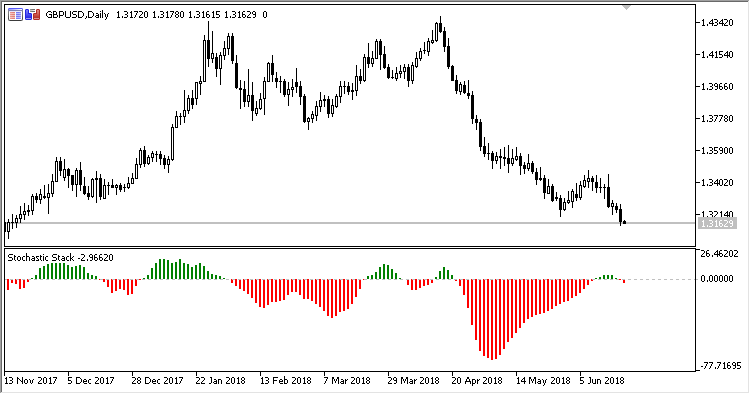Interesting script?
So post a link to it -
let others appraise it

You liked the script? Try it in the MetaTrader 5 terminal# SS - indicator for MetaTrader 5

Views:
1037
Rating:
Published:
2018.07.13 15:21

Indicator SS (Stochastic Stack) represents a set of eight indicators Stochastic with different parameters, united in a single calculation process.

It has forty configurable parameters, five identical parameters for each of the eight stochastics:

• Stoch N %K period - period %K of stochastic N;
• Stoch N %D period - period %D of stochastic N;
• Stoch N slowing - slowing stochastic N;
• Stoch N Method - stochastic N calculation method;
• Stoch N Price field - stochastic N calculation price field.

Calculations:

`SS = (STO1 - STO2) + (STO3 - STO4) + (STO5 - STO6) + (STO7 - STO8)`Translated from Russian by MetaQuotes Software Corp.
Original code: https://www.mql5.com/ru/code/21167RMI

Indicator RMI (Running Median Indicator) is a moving average with the "Running Median" filtering algorithm.PVO

Indicator PVO (Percentage Volume Oscillator) represents a momentum for the volume. It calculates the difference in the percents of large MA between two moving averages based on volumes.UDP

Indicator UDP (Up/Down Percentage) displays the direction of candlesticks, i.e., up/down, as a percentage over the selected period.VFO

The VFO (Volume Flow Oscillator) values above zero are bullish and indicate accumulating, while those below zero indicate distributing. Divergences between the price and the indicator values may indicate a forthcoming change in the trend.